Graphing Quadratic Equations IIT JEE Study Material

Quadratic Equations JEE Study material has been prepared by our experts in the most precise and simplest format covering all the important domains like Quadratic Expressions, Nature of Roots, Condition for common roots, Graphing Quadratic Equations, Quadratic Inequalities, Theory of Equations, etc included in Quadratic Equations JEE Mains and JEE Advanced Syllabus. These IIT JEE study Material could help the students to boost their self-confidence and fetch excellent marks in their IIT JEE and other competitive examinations like BITSAT, VITEEE, etc.

Consider a Quadratic Equation $ax^{2} + bx + c = 0$, where a, b, and c are real and a ≠ 0. The quadratic Equation can be further rewritten as:

$\mathbf{a\left [ \left ( x + \frac{b}{2a} \right )^{2} + \left ( \frac{c}{a} – \frac{b^{2}}{4a^{2}}\right ) \right ]}$

$\mathbf{a\left [ \left ( x + \frac{b}{2a} \right )^{2} + \left ( \frac{4ac – b^{2}}{4a^{2}}\right ) \right ]}$

$\mathbf{a\left [ \left ( x + \frac{b}{2a} \right )^{2} – \left ( \frac{D}{4a^{2}}\right ) \right ]}$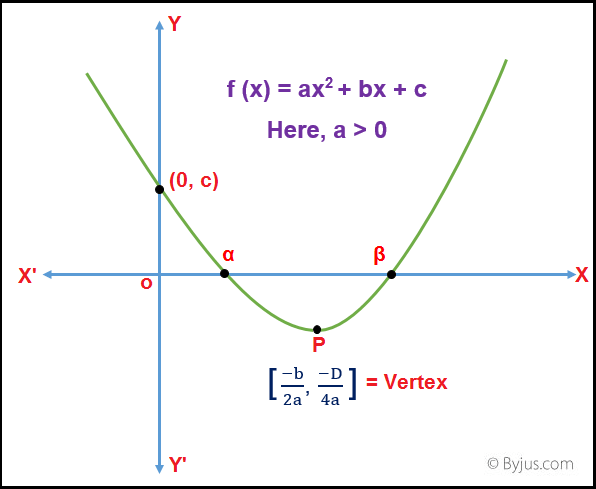Therefore, the above quadratic equation represents a parabola whose vertex is at $\mathbf{P\;\left [ \frac{-b}{2a},\;\frac{-D}{4a}\right ]}$ and axis parallel to y – axis.

In a quadratic Equation the value of ‘a’ determines whether the graph of a quadratic equation will be concave upwards (a > 0) or concave downwards (a < 0). The value of Discriminant ($b^{2} – 4ac$) determines whether the graph of a quadratic Equation will:

1: Intersect the x – axis at two points i.e. $b^{2} – 4ac > 0$,

2: Just touches the x – axis i.e. $b^{2} – 4ac = 0$,,

3: Never intersects the x – axis i.e. $b^{2} – 4ac < 0$

Case 1: When a > 0 and $b^{2} – 4ac > 0$The graph of a quadratic Equation will be concave upwards and will intersect x – axis at two points α and β with α < β. The quadratic Equation will have two real roots (α and β) and the curve will always lie above the x – axis.

1: The quadratic function f(x) will be positive i.e. f(x) > 0, for the values of x lying in the interval $\mathbf{\left ( -\;\infty ,\;\alpha \right )\;\cup \;\left ( \beta ,\;\infty \right )}$

2: The quadratic function f(x) will be Equal to zero i.e. f(x) = 0, if x = α or β

3: The quadratic function f(x) will be negative i.e. f(x) < 0, for the values of x lying in the interval (α, β)

Case 2: When a > 0 and $b^{2} – 4ac = 0$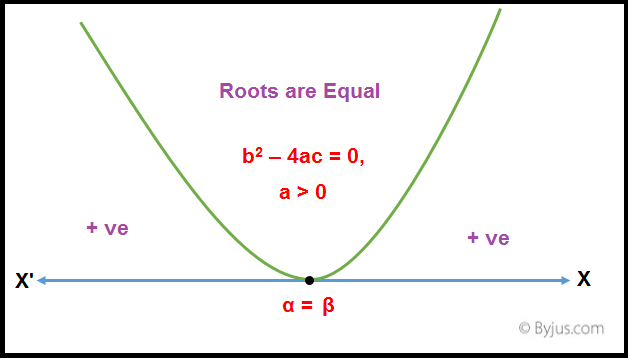The graph of a quadratic Equation will be concave upwards and will touch x – axis at a point $\mathbf{\frac{-b}{2a}}$. The quadratic Equation will have two Equal real roots i.e. α = β. The quadratic function f(x) will be positive i.e. 0 ≤ f(x), $\mathbf{x\;\in R}$

Case 3: When a > 0 and $b^{2} – 4ac < 0$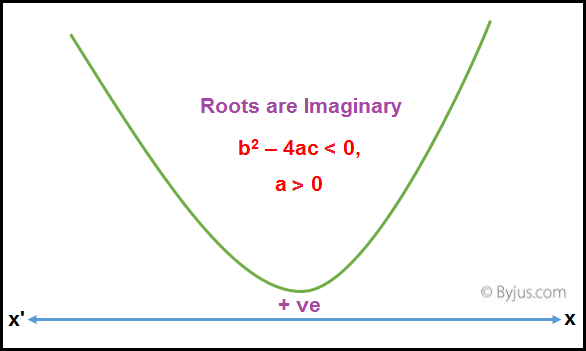The graph of a quadratic Equation will be concave upwards and will not intersect x – axis. The quadratic Equation will have imaginary roots and the curve will always lie above the x – axis. The quadratic function f(x) will be positive i.e. f(x) > 0, $\mathbf{x\;\in R}$

Case 4: When a < 0 and $b^{2} – 4ac > 0$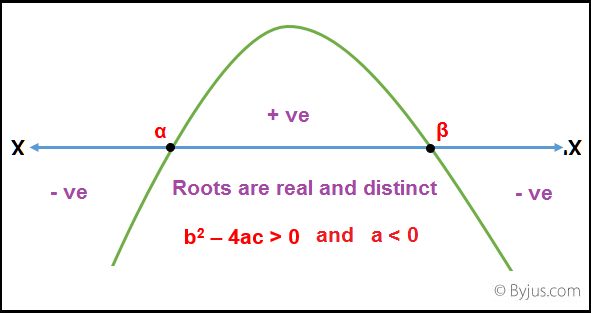The graph of a quadratic Equation will be concave downwards and will intersect x – axis at two points α and β with α < β. The quadratic Equation will have two real roots (α and β) and the curve will always lie below the x – axis.

1: The quadratic function f(x) will be positive i.e. f(x) > 0, for the values of x lying in the interval (α, β).

2: The quadratic function f(x) will be Equal to zero i.e. f(x) = 0, if x = α or β

3: The quadratic function f(x) will be negative i.e. f(x) < 0 for the values of x lying in the interval $\mathbf{\left ( -\;\infty ,\;\alpha \right )\;\cup \;\left ( \beta ,\;\infty \right )}$

Case 5: When a < 0 and $b^{2} – 4ac = 0$The graph of a quadratic Equation will be concave downwards and will touch x – axis at a point $\mathbf{\frac{-b}{2a}}$. The quadratic Equation will have Equal real roots i.e. α = β. The quadratic function f(x) will be negative i.e. f(x) ≤ 0, $\mathbf{x\;\in R}$

Case 6: When a < 0 and $b^{2} – 4ac < 0$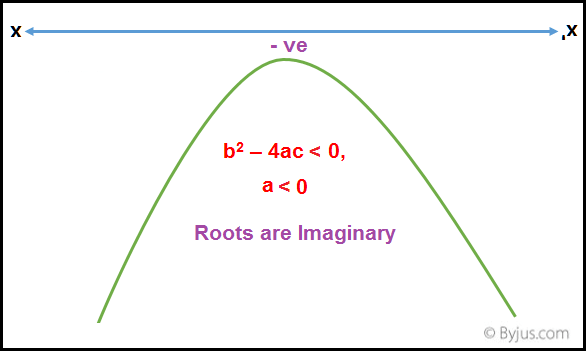The graph of a quadratic Equation will be concave downwards and will not intersect x – axis. The quadratic Equation will have imaginary roots and the curve will always lie below the x – axis. The quadratic function f(x) will be negative i.e. f(x) < 0, $\mathbf{x\;\in R}$

Quadratic Equation IIT JEE Practice Problems

Example 1: If G(x) = $px^{2} + qx + r$ and H(x) = $-px^{2} + mx +r$ and p.r ≠ 0. Prove that G(x) . H(x) = 0 will have exactly two real roots.

Solution:

G(x) will have real roots if $q^{2} – 4pr ≥ 0$ and H(x) will have real roots if $m^{2} + 4pr ≥ 0$.

Case 1: If pr < 0,

$q^{2} – 4pr ≥ 0$, therefore, G(x) will have real root(s).

Case 2: If pr > 0,

$m^{2} + 4pr ≥ 0$, therefore, H(x) will also have real root(s).

Hence at least two roots of G(x) H(x) are real.

Example 2: Find the range in which the value of given quadratic Expression will lie $\mathbf{\frac{x^{2}\;+\;14x\;+\;9}{x^{2}\;+\;2x\;+\;3}}$. [x is a real number]

Solution:

Let, y = $\mathbf{\frac{x^{2}\;+\;14x\;+\;9}{x^{2}\;+\;2x\;+\;3}}$

i.e. $x^{2} + 14x + 9 = (x^{2}y + 2xy + 3y)$

Or, $(1 – y) x^{2} + (7 – y) 2x + 3(3 – y) = 0$

Since x is real, therefore, $b^{2} – 4ac ≥ 0$

i.e. $[2(7 – y)]^{2} – 4[1 – y] \times 3[3 – y] ≥ 0$

Or, $- y^{2} – y + 40 ≤ 0$

Or, (y + 5) (y – 4) ≤ 0

Therefore, the range in which the value of given Quadratic Expression will lie is – 5 ≤ y ≤ 4.

Example 3: Find the values of x for which the equation $\mathbf{\frac{x^{2}\;-\;4x\;+\;3}{x^{2}\;+\;x\;+\;1}\;\leq \;0}$.

Solution:

Let f (x) = $x^{2} – 4x + 3$ and g (x) = $x^{2} + x + 1$.

The coefficient of $x^{2}$ in g (x) is positive and the value of Discriminant (D) < 0. Hence, g(x) is positive for all values of x.

Since, $\mathbf{\frac{f(x)}{g(x)}\;<\; 0}$. Therefore, f (x) must be less than 0.

i.e. $x^{2} – 4x + 3 < 0$

Or, (x – 3) (x – 1) < 0

Therefore, 1 < x < 3.

Practise This Question

The exchange of incoming and outgoing radiations that warms the Earth is called ___.